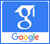# Gamma-ray spectrometry## Using Mathematical Procedure to Compute the Attenuation Coefficient in Spectrometry Field

Published on: 6th February, 2017

OCLC Number/Unique Identifier: 7286351538

In gamma-ray spectrometry, the analysis of the environmental radioactivity samples (soil, sediment and ash of a living organism) needs to know the linear attenuation coefficient of the sample matrix. This coefficient is required to calculate the self-absorption correction factor through the sample bulk. In addition, these parameters are very important because the unidentified samples can be different in the composition and density from the reference liquid sources which are usually used for efficiency calibration in the radioactive monitoring process. The present work is essentially concerned to introduce a mathematical method to calculate the linear attenuation coefficient without using any collimator. This method was based mainly on the calculations of the effective solid angle subtended by the source-to-the detector configurations, the efficiency transfer technique and the average path lengths through the samples itself. The method can be used as a tool for the calculation of the linear attenuation coefficient of unidentified materials with good facility to use it in the calibration process of γ-ray detectors, particularly in the study of soil samples. The results are compared with the data from NIST-XCOM to show how much the results are in close agreement and to give the validity of the approach.